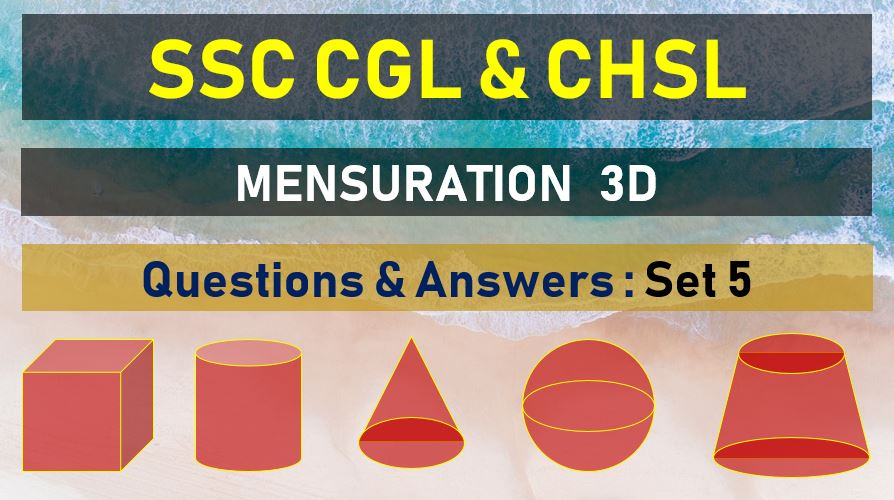Tuesday, June 22, 2021
Home > mensuration > SSC CGL Surface Area and Volume Questions Answers Set 5

# SSC CGL Surface Area and Volume Questions Answers Set 5SSC Mensurations 3D
Surface Area & Volume Questions Sets
Set 1Set 2Set 3Set 4Set 5
Set 6Set 7Set 8Set 9Set 10

SSC CGL Surface Area and Volume Questions Answers Set 5: Ques No 1

The base of a right prism is a right angled triangle whose sides are 5 cm, 12 cm and 13 cm. If the total surface area of the prism is 360 cm2, then its height (in cm) is

Options:

A. 10

B. 12

C. 9

D. 11

Answer: A

Solution:

SSC CGL Surface Area and Volume Questions Answers Set 5: Ques No 2

A right pyramid stands on a square base of side 16 cm, and its height is 15 cm. The area (in cm2) of its slant surface is

Options:

A. 514

B. 544

C. 344

D. 444

Answer: B

Solution:

SSC CGL Surface Area and Volume Questions Answers Set 5: Ques No 3

If the altitude of a right prism is 10 cm and its base is an equilateral triangle of side 12 cm, then its total surface area (in cm2) is

Options:

A. (5+ 3√3)

B. 36√3

C. 360

D. 72(5+√3)

Answer: D

Solution:

SSC CGL Surface Area and Volume Questions Answers Set 5: Ques No 4

A right pyramid stands on a square base of diagonal 10√2 cm. If the height of the pyramid is 12 cm, the area (in cm2) of its slant surface is

Options:

A. 520

B. 420

C. 360

D. 260

Answer: D

Solution:

SSC CGL Surface Area and Volume Questions Answer Set 5: Ques No 5

A right prism stands on a base of 6 cm side equilateral triangle and its volume is 81√3 cm3. the height (in cm ) of the prism is

Options:

A. 9

B. 10

C. 12

D. 15

Answer: A

Solution:

SSC CGL Surface Area and Volume Questions Answer Set 5: Ques No 6

The base of a right pyramid is equilateral triangle of side 10√3 cm. if the total surface are of the pyramid is 270√3 cm2. Its height is

Options:

A. 12√3 cm

B. 10 cm

C. 10√3 cm

D. 12 cm

Answer: D

Solution:

SSC CGL Surface Area and Volume Questions Answer Set 5: Ques No 7

A sphere, a cylinder and a cone are of the same radius and same height. Find the ratio of their curved surfaces

Options:

A. 4 : 4 : √5

B. 4 : 5 : √5

C. 3 : 4 : √5

D. 2 : 3 : √5

Answer: A

Solution:

SSC CGL Surface Area and Volume Questions Answer Set 5: Ques No 8

The base of right prism is a triangle whose perimeter is 28 cm and the in radius of the triangle is 4 cm. If the volume of the prism is 366 cc, then its height is

Options:

A. 6 cm

B. 8 cm

C. 4 cm

D. None of these

Answer: D

Solution:

SSC CGL Surface Area and Volume Questions Answer Set 5: Ques No 9

Three equal cubes are placed adjacently in a row. Find the ratio of the total surface area of the new cuboid to that of the sum of the surface area of those cubes.

Options:

A. 5 : 9

B. 3 : 5

C. 2 : 5

D. 7 : 9

Answer: D

Solution:

SSC CGL Surface Area and Volume Questions Answer Set 5: Ques No 10

The length of each edge of a regular tetrahedron is 12 cm. The area (in sq. cm) of the total surface of the tetrahedron is

Options:

A. 288√3

B. 144√2

C. 108√3

D. 144√3

Answer: D

Solution:

SSC Mensurations 3D
Surface Area & Volume Questions Sets
Set 1Set 2Set 3Set 4Set 5
Set 6Set 7Set 8Set 9Set 10
error: Content is protected !!